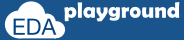## \$urandom( )

The system function \$urandom provides a mechanism for generating pseudorandom numbers. The function returns a new 32-bit random number each time it is called. The number shall be unsigned.

```variable = \$urandom(seed);
```

The seed is an optional argument that determines the sequence of random numbers generated. The seed can be an integral expression. for a particular seed, the same value will get generated.

```bit [31:0] addr1;
bit [31:0] data;
```

```addr1 = \$urandom();
data  = \$urandom * 6;
```

## \$random( )

\$random() is same as \$urandom() but it generates signed numbers.

## \$urandom_range( )

The \$urandom_range() function returns an unsigned integer within a specified range.

```\$urandom_range( int unsigned maxval, int unsigned minval = 0 );
```

```addr1 = \$urandom_range(30,20);
addr2 = \$urandom_range(20);    //takes max value as '0'
addr3 = \$urandom_range(20,30); //considers max value as '30' and min value as '20'
```

## random system methods examples

In the example below,
Shows usage of \$urandom and \$urandom_range.

```module system_funcations;
bit [31:0] data;
```

```  initial begin
data  = \$urandom * 6;

```    \$display("addr1=%0d, addr2=%0d, addr3=%0d",addr1,addr2,addr3);
Execute the above code on